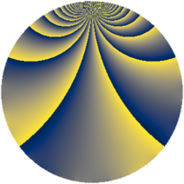# Properties

 Label 585.2.bfLevel $585$ Weight $2$ Character orbit 585.bf Rep. character $\chi_{585}(199,\cdot)$ Character field $\Q(\zeta_{6})$ Dimension $64$ Newform subspaces $3$ Sturm bound $168$ Trace bound $1$

# Related objects

## Defining parameters

 Level: $$N$$ $$=$$ $$585 = 3^{2} \cdot 5 \cdot 13$$ Weight: $$k$$ $$=$$ $$2$$ Character orbit: $$[\chi]$$ $$=$$ 585.bf (of order $$6$$ and degree $$2$$) Character conductor: $$\operatorname{cond}(\chi)$$ $$=$$ $$65$$ Character field: $$\Q(\zeta_{6})$$ Newform subspaces: $$3$$ Sturm bound: $$168$$ Trace bound: $$1$$ Distinguishing $$T_p$$: $$2$$

## Dimensions

The following table gives the dimensions of various subspaces of $$M_{2}(585, [\chi])$$.

Total New Old
Modular forms 184 72 112
Cusp forms 152 64 88
Eisenstein series 32 8 24

## Trace form

 $$64 q - 30 q^{4} + O(q^{10})$$ $$64 q - 30 q^{4} + 2 q^{10} + 12 q^{11} - 20 q^{14} - 14 q^{16} + 12 q^{19} + 6 q^{20} + 4 q^{25} - 34 q^{26} + 24 q^{29} - 4 q^{35} - 56 q^{40} + 36 q^{41} - 54 q^{46} - 28 q^{49} - 42 q^{50} + 10 q^{55} + 68 q^{56} - 24 q^{59} + 20 q^{61} + 24 q^{64} + 4 q^{65} - 24 q^{71} + 2 q^{74} - 90 q^{76} - 16 q^{79} - 84 q^{80} + 4 q^{91} - 8 q^{94} - 6 q^{95} + O(q^{100})$$

## Decomposition of $$S_{2}^{\mathrm{new}}(585, [\chi])$$ into newform subspaces

Label Dim $A$ Field CM Traces $q$-expansion
$a_{2}$ $a_{3}$ $a_{5}$ $a_{7}$
585.2.bf.a $8$ $4.671$ 8.0.49787136.1 None $$0$$ $$0$$ $$0$$ $$0$$ $$q+(\beta _{1}+\beta _{5})q^{2}+(\beta _{2}+\beta _{4}+\beta _{6})q^{4}+\cdots$$
585.2.bf.b $24$ $4.671$ None $$0$$ $$0$$ $$0$$ $$0$$
585.2.bf.c $32$ $4.671$ None $$0$$ $$0$$ $$0$$ $$0$$

## Decomposition of $$S_{2}^{\mathrm{old}}(585, [\chi])$$ into lower level spaces

$$S_{2}^{\mathrm{old}}(585, [\chi]) \cong$$ $$S_{2}^{\mathrm{new}}(65, [\chi])$$$$^{\oplus 3}$$$$\oplus$$$$S_{2}^{\mathrm{new}}(195, [\chi])$$$$^{\oplus 2}$$